# Texas Go Math Grade 3 Lesson 5.2 Answer Key Use Place Value to Subtract

Refer to our Texas Go Math Grade 3 Answer Key Pdf to score good marks in the exams. Test yourself by practicing the problems from Texas Go Math Grade 3 Lesson 5.2 Answer Key Use Place Value to Subtract.

## Texas Go Math Grade 3 Lesson 5.2 Answer Key Use Place Value to Subtract

Unlock the Problem

Ava sold 473 tickets for the school play. Kim sold 294 tickets. How many more tickets did Ava sell than Kim?

Do you need to combine or compare the number of tickets sold?
Ava sold 179 more tickets.
We compare the number of tickets sold.
Explanation:
Ava sold 473 tickets.
Kim sold 294 tickets.
By comparing the tickets sold by both of them, Ava sold more.

Circle the numbers you will need to use.Explanation:
Circle the number of tickets sold by Ava i.e., 473 tickets.
Circle the number of tickets sold by Kim i.e., 294 tickets.

Use place value to subtract.

Subtract. 473 – 294
Estimate. 475 – 300 = __________

STEP 1:
Subtract the ones.
3 < 4, so regroup.
7 tens 3 ones =
6 tens ________ onesSTEP 2:
Subtract the tens.
6 < 9, so regroup.
4 hundreds 6 tens =
3 hundreds ________ tensSTEP 3:Addition and subtraction are opposite operations, or inverse operations. So you can use addition to check subtraction.

So, Ava sold Since ___________ more tickets than Kim.
Since _________ is close to the estimate of __________, the answer is reasonable.
Ava sold Since 179 more tickets than Kim.
Since 179  is close to the estimate of 180 the answer is reasonable.
Explanation:
Ava sold 473 tickets.
Kim sold 294 tickets.
By comparing the tickets sold by both of them, Ava sold more.

Math Talk
Mathematical Processes

Rounding the number to nearer to ten or hundred to get a simple number.

Try This! Circle the problem in which you need to regroup. Find the difference.

A.Explanation:
521 – 301 = 220
No need to regroup, because the difference is nearer to its ten.

B.Explanation:
This problem to be circled
918 – 427 = 491
Regrouping to be done as shown below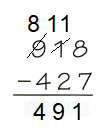Share and Show

Estimate. ________ – _______ = __________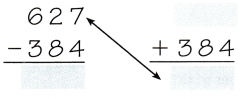Since ________ is close to the estimate of ________, the answer is reasonable.
Estimate. 625 – 385  = _240__Since  243 is close to the estimate of  240  the answer is reasonable.

Math Talk
Mathematical Processes

Did you need to regroup to find the difference? Explain.
Explanation:
Line up the tens place and the ones place and get ready to subtract the ones.
But you cant subtract 9 from 7 because 9 is bigger than 7.
So you have to take one group of 10 (ten 1s) from the tens place.
Now you have 7 groups of ten and seventeen 1s.
You’ve regrouped the 87 into different sized groups.
If subtraction between the smaller to bigger number
Example 9 – 7
Regrouping to be done

Estimate. Then find the difference.

Question 2.
Estimate: _________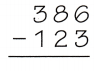Estimate: 260
Explanation:
385 – 125 = 260Since  263 is close to the estimate of  260  the answer is reasonable.

Question 3.
Estimate: __________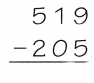Estimate: 310
Explanation:
520 – 200 = 320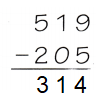Since  314 is close to the estimate of  310  the answer is reasonable.

Go Math Lesson 5.2 Place Value Answer Key 3rd Grade Question 4.
Estimate: ___________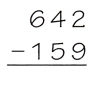Estimate: 480
Explanation:
640 – 160 = 480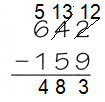Since  483 is close to the estimate of  480  the answer is reasonable.

Question 5.
Estimate: ___________Estimate: 20
Explanation:
240 – 220 = 20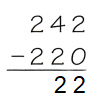Since  22 is close to the estimate of  20  the answer is reasonable.

Math Talk
Mathematical Processes

Which exercises can you compute mentally? Explain why.
Using place value to subtract.
Explanation:
STEP 1:
Subtract the ones, tens, hundreds and so on,
first regroup and estimate the answer.
For example:
3 < 4, so regroup.
7 tens 3 ones =
6 tens ________ onesProblem Solving

H.O.T. Algebra Find the unknown number.

Question 6.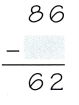Explanation:
n is unknown number
86 – n = 62
86 – 62 = n
n = 24

Question 7.Explanation:
372 – n = 240
372 – 240 = n
n = 132

Texas Go Math Grade 3 Answer Key Pdf Lesson 5.2 Question 8.Explanation:
537 – n = 169
537 – 169 = n
n = 368

Question 9.Explanation:

629 – n = 335
629 – 335 = n
n = 294

Question 10.
The Dessert Store bought 120 peaches. The bakers made peach jam and peach pies with 98 of the peaches. How many peaches are left to make peach cobbler?
22 peaches left to make cobbler
Explanation:
The Dessert Store bought 120 peaches.
The bakers made peach jam and peach pies with 98 of the peaches.
Number of peaches left
120 – 98 = 22

Question 11.
Multi-Step The stamp store has 857 stamps for sale. They sold 156 stamps on Monday and 348 stamps on Tuesday. How many stamps do they have left for sale on Wednesday?
353 left for sale on Wednesday
Explanation:
The stamp store has 857 stamps for sale.
They sold 156 stamps on Monday and 348 stamps on Tuesday.
Number of stamps left for sale on Wednesday
156 + 348 = 504
857 – 504 = 353

Problem Solving

Use the table for 12-13.Question 12.
How many more tickets did Sonja sell than Matt?
153 more tickets did Sonja sell than Matt
Explanation:
Sonja sold 331 tickets.
Matt sold 178 tickets.
By comparing both Sonja sold more.
331 – 178 = 153

H.O.T. Multi-Step Alicia sold 59 fewer tickets than Jenna and Matt sold together. How many tickets did Alicia sell? Explain.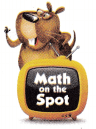223 tickets.
Explanation:
Alicia sold 59 fewer tickets than Jenna and Matt sold together.
We know from the given table Jenna sold 282 tickets.
Matt sold 178 tickets.
Number of tickets sold by Alicia
282 – 59 = 223

Question 14.
H.O.T. Sense or Nonsense? Nina says to check subtraction, add the difference to the number you started with. Does this statement make sense? Justify.
Addition is used to check for subtraction errors by adding the answer we find to the bottom number in the problem.
Explanation:Question 15.
Use Math Language Do you have to regroup to find 523 – 141? Explain. Then solve.
YES, Regroup is to be done
Explanation: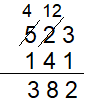Question 16.
The company has 228 large water bikes and 136 small water bikes. How many more large water bikes does the company have than small water bikes?
(A) 92
(B) 94
(C) 112
(D) 191
Option (A)
Explanation:
The company has 228 large water bikes and 136 small water bikes.
Number of large water bikes the company has than small water bikes
228 – 136 = 92

A store has 448 blankets and 607 pillows. How many fewer blankets does the store have than pillows?
(A) 168
(B) 159
(C) 161
(D) 249
Option (B)
Explanation:
A store has 448 blankets and 607 pillows.
Number of fewer blankets  the store have than pillows
607 – 448 = 159

Question 18.
Multi-Step Hannah, Sam, and Alex play a game. Hannah has 325 points. Sam has 234 points. Alex has 124 fewer points than Hannah and Sam together. How many points does Alex have?
(A) 683
(B) 559
(C) 435
(D) 201
Option (C)
Explanation:
Hannah has 325 points.
Sam has 234 points.
Alex has 124 fewer points than Hannah and Sam together.
Number of points Alex has
325 + 234 = 559
559 – 124 =435

Texas Test Prep

Question 19.
There are 842 seats in the school auditorium. 138 seats need repairs. How many seats do not need repairs?
(A) 804
(B) 980
(C) 716
(D) 704
Option (D)
Explanation:
There are 842 seats in the school auditorium.
138 seats need repairs.
Number of seats do not need repairs
842 – 138 = 704

### Texas Go Math Grade 3 Lesson 5.2 Homework and Practice Answer Key

Estimate. Then find the difference.

Question 1.
Estimate: _________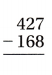Estimate: 260
Explanation:
rounding the 427 to 430 and 168 to 170
estimate is 260
430 – 170 = 260

Estimate: _________Estimate: 610
Explanation:
rounding the 846 to 850 and 239 to 240
850 – 240 = 610

Question 3.
Estimate: __________Estimate: 350
Explanation:
780 – 430 = 350

Use the table for 4-6.Question 4.
About how many more black bears than grizzly bears are at Yellowstone Park?
Explanation:
Number of black bears = 729
Number of grizzly bears = 483
By comparing both, black bears are more.
729 – 483 = 246

Question 5.
How many more black bears are there than brown bears at the park?
Explanation:
Number of black bears = 729
Number of brown bears = 381
By comparing both, black bears are more.
729 – 381 = 348

Question 6.
How many more grizzly and brown bears are there than black bears?
Explanation:
Number of brown bears = 381
Number of grizzly bears = 483
By comparing both, grizzly bears are more.
483 – 381 = 102

Problem Solving

Question 7.
Fiona subtracts 617 – 234 = 483. Is her answer correct? Explain.
Explanation:
When we subtract the difference is more than 100 than the actual sum.
617 – 234 = 383

Byron’s Math Club wants to sell 500 boxes of granola bars. So far, they have sold 287 boxes. How many more boxes do they need to sell?
Explanation:
Byron’s Math Club wants to sell 500 boxes of granola bars.
So far, they have sold 287 boxes.
Number of boxes need to sell
500 – 287 = 213

Lesson Check

Question 9.
There are 526 students at the New City Elementary School. Of these, 178 are in the third grade. How many students are not in the third grade?
(A) 448
(B) 348
(C) 358
(D) 458
Option (B) 348
Explanation:
There are 526 students at the New City Elementary School.
Of these, 178 are in the third grade.
Number of students not in grade 3
526 – 178 = 348

Question 10.
In the morning, there are 472 visitors at the Street Fair. In the afternoon there are 218 visitors. About how many more visit the fair in the morning?
Option (A)
Explanation:
There are 472 visitors at the Street Fair.
In the afternoon there are 218 visitors.
Number of visitors in morning
472 – 218 = 254
ESTIMATE : 470 – 220 = 250

Question 11.
The third grade collects 417 bottles to recycle. The fourth grade collects 378 bottles. How many more bottles does the third grade collect?
(A) 49
(B) 139
(C) 39
(D) 149
Option (C)
Explanation:
The third grade collects 417 bottles to recycle.
The fourth grade collects 378 bottles.
417 – 378 = 39

Question 12.
A museum store has 239 posters, 125 calendars, and 547 books. How many more books than posters does the museum store have?
(A) 114
(B) 312
(C) 422
(D) 308
Option (D)
Explanation:
A museum store has 239 posters, 125 calendars, and 547 books.
Number of books more than poster
547 – 239 = 308

Multi-Step Darius has 127 photos. Miquel has 179 photos and Jillian has 467 photos. How many more photos does Lillian have than both Darius and Miquel?
(A) 773
(B) 171
(C) 261
(D) 161
Option (D)
Explanation:
Darius has 127 photos.
Miquel has 179 photos.
Jillian has 467 photos.
The number of photos Jillian has than both Darius and Miquel
127 + 179 = 306
467 – 306 = 161

Question 14.
Multi-Step Beth, Dan, and Yoshi collect stamps. Beth has 157 stamps. Dan has 265 stamps. Yoshi has 79 fewer stamps than Beth and Dan. How many stamps does Yoshi have?
(A) 343
(B) 333
(C) 243
(D) 233